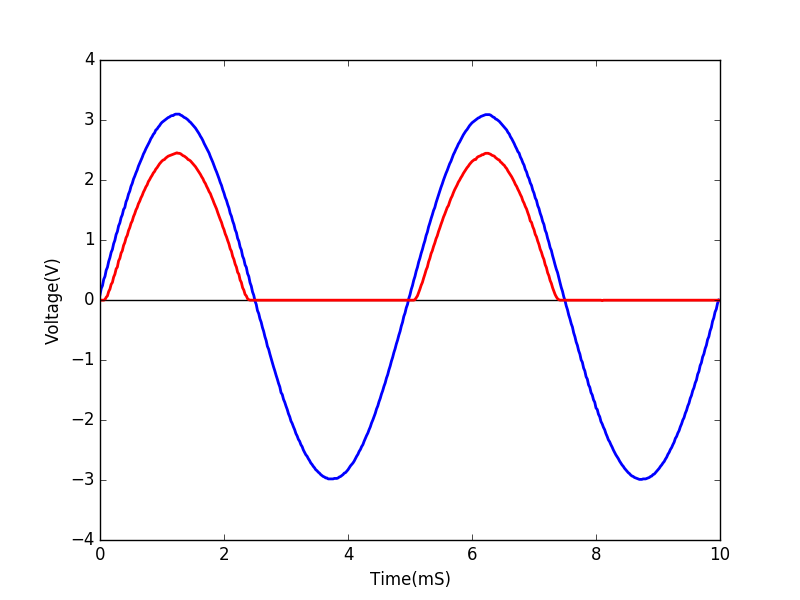# Half-Wave Rectification

## Study a halfwave rectifier using a 4148 diode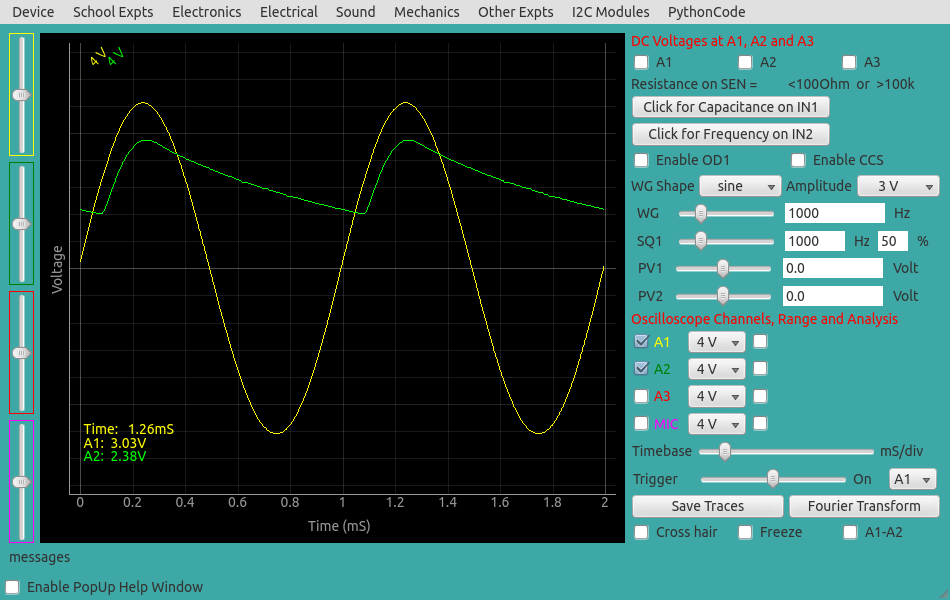Brief Summary
Study half-wave rectifiers With ExpEYES. All you need is the waveform generator and oscilloscope of ExpEYES, and a few passive components such a diode, capacitor, and resistor.

## Introduction

Half wave rectifiers cut off one half of a waveform, and further filtering with a capacitor enables generation of a DC signal from an AC signal.

## Quick Start

The waveform generator WG is set to give a sine wave of 1kHz. It is monitored by oscilloscope channel A1. The signal after the diode is monitored by A2. The observed waveform will be a bit noisy without the load resistor, connecting a 1k resistor gives a clean rectified waveform. The voltage drop across the diode is clearly visible. Connect different values of capacitors to view the filtering effect.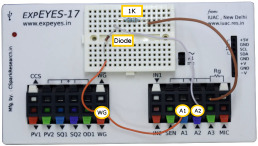## Screenshot of the UI

Screen shot of the oscilloscope program showing input and output of half wave rectifier. 1N4148 diode at 1000Hz and 1kOhm load resistor.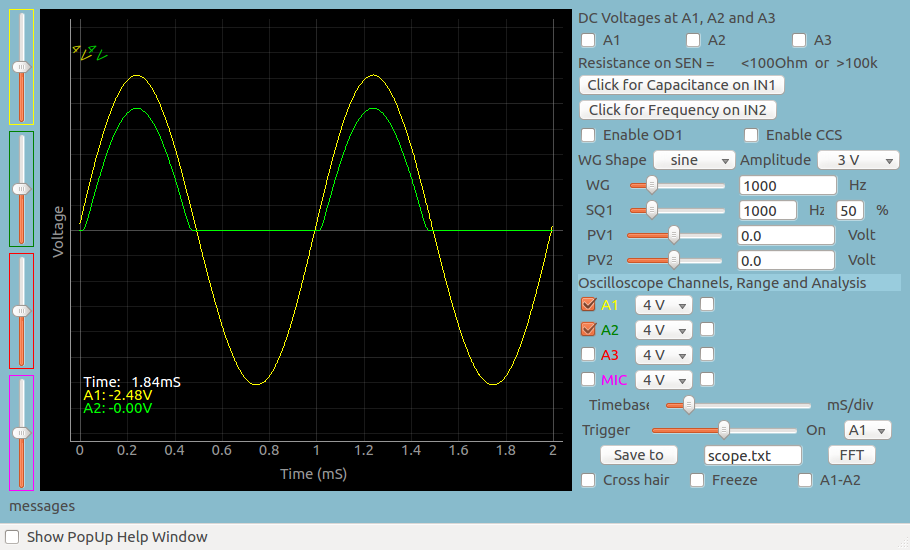### Drop in the peak voltage

In case you hadn’t noticed, the output waveform has a lower peak voltage than the input. This is a result of the barrier potential

### Exercises

• Measure the drop in peak voltage for different diodes ( 4148, LED, Zener, Schottky…)
• Correlate your measurements with the knee voltage measured with the Diode IV characteristics experiment

## Filtering with a 1uF Capacitor

The output after adding a 1uF capacitor for filtering is shown below. With increasing RC value, the ripple reduces.## Junction capacitance

Every diode has a junction capacitance that acts like a capacitance connected in parallel to the ideal PN junction. Junction capacitance of 1N4148 is only 4pF but 1N4007 has a junction capacitance of 20pF. The rectified output, without external load resistor, for 1N4007 is shown below. The 1MOhm input impedance of channel A2 will be always present.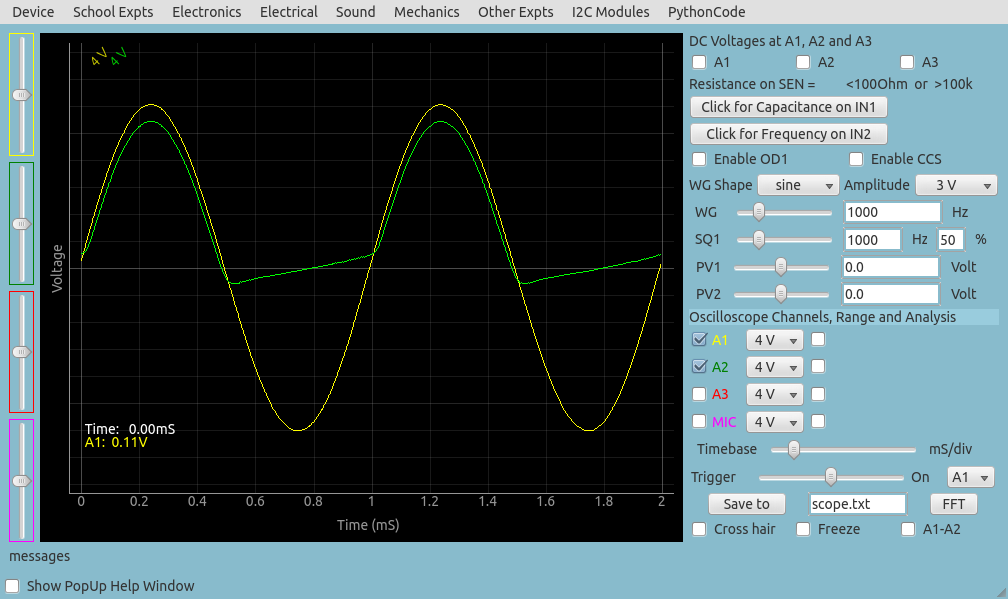## Write Python Code

This experiment can also be done by running this Python Code. The output of the program is shown below.

``````#Python code to study a halfware rectifier
import eyes17.eyes
p = eyes17.eyes.open()

from pylab import *

p.set_sine(200)
t,v, tt,vv = p.capture2(500, 20)   # captures A1 and A2

xlabel('Time(mS)')
ylabel('Voltage(V)')
plot([0,10], [0,0], 'black')
ylim([-4,4])
plot(t,v,linewidth = 2, color = 'blue')
plot(tt, vv, linewidth = 2, color = 'red')
show()
``````

### Output of the code